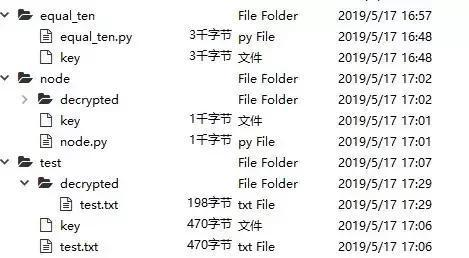### 用 Python 加密文件，技能满满，赶紧get起来

• 一位二进制数与自身的异或值为 0

b ^ b = 0

• 异或操作满足交换律

a ^ b ^ c = a ^ (b ^ c) = (a ^ b) ^ c

• 0 与 a 的异或为 a

(a ^ b) ^ b = a ^ (b ^ b) = a ^ 0 = a\

secrets 库是 Python 3.6 引入的伪随机数模块，适合生成随机密钥。token_bytes 函数接受一个 int 参数，用于指定随机字节串的长度。int.from_bytes 把字节串转换为 int，也就是我们需要的二进制数。

from secrets import token_bytes
def random_key(length):
key = token_bytes(nbytes=length)
key_int = int.from_bytes(key, ‘big’)
return key_int\

encrypt 函数接受一个 str 对象，返回元组 (int, int)。通过 encode 方法，我们将字符串编码成字节串。int.from_bytes 函数将字节串转换为 int 对象。最后对二进制对象和随机密钥进行异或操作，就得到了加密文本。

def encrypt(raw):
raw_bytes = raw.encode()
raw_int = int.from_bytes(raw_bytes, ‘big’)
key_int = random_key(len(raw_bytes))
return raw_int ^ key_int, key_int\

decrypt 接受两个 int 对象，分别为加密文本和随机密钥。首先对两者进行异或操作，计算解密出来的 int 对象所占比特数。decrypted.bit_length 函数得到的是二进制数的位数，除以 8 可以得到所占比特大小。为了防止，1 ~ 7 位的二进制数整除 8 得到 0，所以要加上 7，然后再进行整除 8 的操作。使用 int.to_bytes 函数将解密之后的 int 的对象转换成 bytes 对象。最后通过 decode 方法，将字节串转换成字符串。最后，如果你的时间不是很紧张，并且又想快速的python提高，最重要的是不怕吃苦，建议你可以价位@762459510 ，那个真的很不错，很多人进步都很快，需要你不怕吃苦哦！大家可以去添加上看一下~

def decrypt(encrypted, key_int):
decrypted = encrypted ^ key_int
length = (decrypted.bit_length() + 7) // 8
decrypted_bytes = int.to_bytes(decrypted, length, ‘big’)
return decrypted_bytes.decode()\

raw = ‘画图省识春风面，环珮空归夜月魂’
encrypted = encrypt(raw)
encrypted
(217447100157746604585…,
9697901906831571319…)\

decrypt(*encrypted)
‘画图省识春风面，环珮空归夜月魂’\

path 为待加密文件的地址，如果不指定密钥地址，则在该目录下新建目录和文件。

import json
from pathlib import Path
def encrypt_file(path, key_path=None, *, encoding=‘utf-8’):
path = Path(path)
cwd = path.cwd() / path.name.split(’.’)
path_encrypted = cwd / path.name
if key_path is None:
key_path = cwd / ‘key’
if not cwd.exists():
cwd.mkdir()
path_encrypted.touch()
key_path.touch()
with path.open(‘rt’, encoding=encoding) as f1,
path_encrypted.open(‘wt’, encoding=encoding) as f2,
key_path.open(‘wt’, encoding=encoding) as f3:
json.dump(encrypted, f2)
json.dump(key, f3)\

def decrypt_file(path_encrypted, key_path=None, *, encoding=‘utf-8’):
path_encrypted = Path(path_encrypted)
cwd = path_encrypted.cwd()
path_decrypted = cwd / ‘decrypted’
if not path_decrypted.exists():
path_decrypted.mkdir()
path_decrypted /= path_encrypted.name
path_decrypted.touch()
if key_path is None:
key_path = cwd / ‘key’
with path_encrypted.open(‘rt’, encoding=encoding) as f1,
key_path.open(‘rt’, encoding=encoding) as f2,
path_decrypted.open(‘wt’, encoding=encoding) as f3: